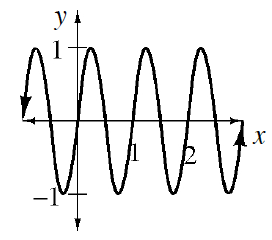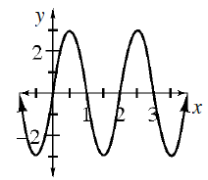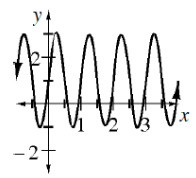### Home > INT3 > Chapter 10 > Lesson 10.1.2 > Problem10-34

10-34.

Sketch the graph of each equation below.

For each of the following, determine the amplitude, period, and vertical shift.

1. $y = \sin\left(2πx\right)$

This is the graph of $y = \sin x$ with a changed period.2. $y = 3\sin\left(πx\right)$3. $y = 2\sin\left(2πx\right) + 1$